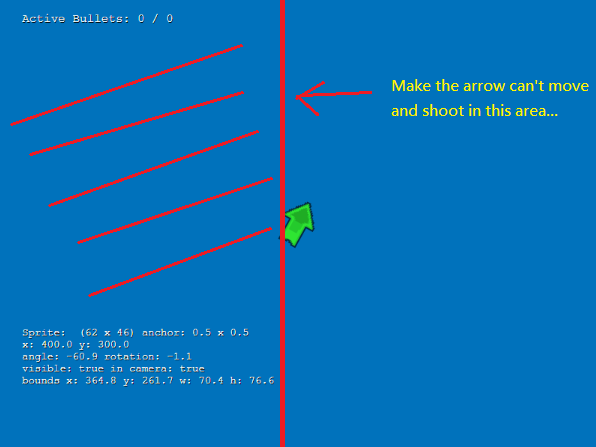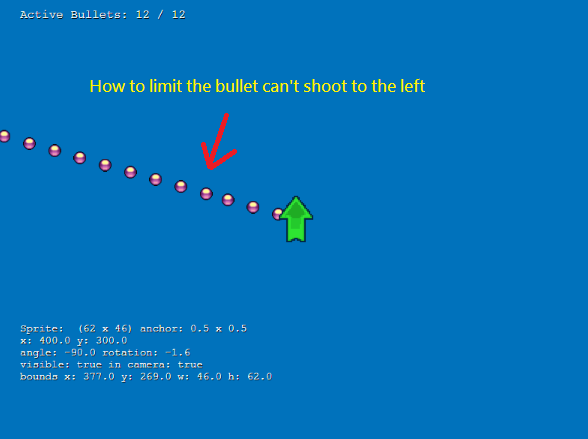# [Solved]How to limit the shooting direction of the sprite??

## Recommended Posts

I use the following example to get start.

When the arrow's angle  <= 90 and  >= 90, the arrow will stop move and can not shoot to the left!!!!``````function update() {
//right
if(sprite.angle <= -90 && sprite.angle >= -180){
sprite.angle=-90;
} else if(sprite.angle >= 90 && sprite.angle <= 180){
sprite.angle=90;
}
//left
/*
if(sprite.angle >= -90 && sprite.angle <= 0){

sprite.angle = -90;

} else if(sprite.angle <= 90 && sprite.angle >= 0){
sprite.angle = 90;
}

*/
}
``````

But  I hope the bullet not shoot to the left, any idea??? Thanks you so muchHere is the jsfiddle link, Thanks

##### Share on other sites

You need to add the same angle constraints to bullet firing too.

So instead of firing when the pointer is down,

``````if (game.input.activePointer.isDown){
fire();
}    ``````

you fire when the pointer is down and your sprite follows your angle constraints.

``````if (game.input.activePointer.isDown && sprite.angle >= -90 && sprite.angle <=90){
fire();
}    ``````

##### Share on other sites

1 hour ago, gauravD said:

You need to add the same angle constraints to bullet firing too.

So instead of firing when the pointer is down,

```
```if (game.input.activePointer.isDown){
fire();
}    ``````

you fire when the pointer is down and your sprite follows your angle constraints.

```
```if (game.input.activePointer.isDown && sprite.angle >= -90 && sprite.angle <=90){
fire();
}    ``````

Works perfectly!!! You are so awesome!!!Thank you very much and have a nice day!!